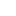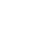# Estimation of the amount of nickel by back titration

Estimation of the amount of nickel by back titration

#### Chemicals used:

• Nickel sample solution
• EDTA
• Eriochrome Black T
• Ammonia buffer
• Zinc acetate as a standard solution
• water

#### Apparatus required:

• Burette
• Pipette
• Measuring cylinder
• Beaker
• Iron stand

#### Theory:

Back titration

Back titration is a type of titration method where the concentration of an analyte is determined by reacting it with a known amount of excess of reagent is known as back titration. The remaining excess reagent is then titrated with another standard solution. It is a type of complexometric titration. Back titration or indirect titration is usually a two-stage technique.

• The reaction between the substances of interest with a known amount of a reactant in excess
• Then the remaining standard solution is titrated with another solution

In a direct titration then we add the standard solution to the analyte until we reach the endpoint, while in the case of back titration we add an excess standard solution then titrate it with another solution. For example in the case of zinc analysis, we use zinc acetate as a primary standard this titration gives the sharp point.

#### Importance:

Back titration is also useful if the reaction between an analyte and titrant is very low at room temperature. If the analyte and titrant do not react then we can also use the same technique.

#### Indicator:

A substance that causes color change at an equivalence point when certain pH is gained is known as an indicator. The indicator is pH-dependent species. Eriochrome Black T is used as an indicator in complexometric titration in which it works in basic PH 10.

#### Endpoint:

In the case of the back titration, the endpoint is opposite to the direct titration, which is blue to wine red.

Estimation of the amount of nickel by back titration

#### Procedure:

• First of all was washed and cleaned carefully and write properly
• 10 ml of sample solution i.e. nickel sample was taken in a conical flask with the help of a pipette
• Excess of EDTA solution i.e. near about 20 ml was taken with the help of a measuring cylinder and poured into the conical flask
• 1-2 ml of buffer solution of PH 10 was added to the conical flask with the help of a measuring cylinder
• A few crystals of EBT were added in the conical flask and the color of the solution became blue and It was shacked well for 5 minutes
• Now it was titrated against a standard solution of zinc acetate taken in the burette
• The point where the colour of solution became wine was noted was noted
• The same procedure was repeated two to three times to get three readings

#### Observation and calculations:

The volume of EDTA solution was taken = 20 ml

Molarity of EDTA solution = 0.01 m

 Sr. No Initial volume Final volume Volume used 1 0.0 10.8 10.8 2 10.8 21.5 10.7 3 21.5 32.1 10.6

Mean volume = 10.8 +10.7+10.6/3

= 10.7ml

The volume of EDTA used for Ni = 20 – 10.7

= 9.3 ml

By dilution formula

Standard solution = Sample solution

M1V1 = M2V2

1. × 9.3 = M2 × 10

M2 = 0.0093

Now we can find the strength of Ni sample

Strength per liter =molarity × mol. mass

= 0.0093 × 58.69

= 0.545 g /L

#### Precautions:

• Glassware should be washed and dried carefully
• Burette should not touch the sides of the conical flask
• Avoid direct touching the chemicals
• Always use measuring cylinder for buffer solution because of fumes of ammonia

#### Result:

The strength of nickel was 0.545 gram per liter

Estimation of the amount of nickel by back titration###### Don’t miss these Chemistry topics!
Sharing Is Caring:

### 13 thoughts on “Estimation of the amount of nickel by back titration”

1.Excellent work.

2.Very informative

3.Nice work

•thank you

4.Great attempt

5.Good job

•thanks

6.Very informative

•thanks

7.Very nice

8.Nice work
I wish you all the best

•9.